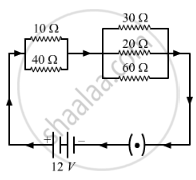# In the Circuit Diagram Given Below Five Resistances of 10 ω, 40 ω, 30 ω, 20 ω, and 60 ω Are Connected as Shown to a 12 V Battery. - Science

In the circuit diagram given below five resistances of 10 Ω, 40 Ω, 30 Ω, 20 Ω, and 60 Ω are connected as shown to a 12 V battery.Calculate:
(a) total resistance in the circuit.

(b) total current flowing in the circuit.

#### Solution

(a) When three resistors are connected in parallel, the net resistance can be obtained as followed:
1/R = 1/R1 + 1/R2 + 1/R3
The resistors of 30 Ω, 20 Ω and 60 Ω are connected in parallel. Therefore, the net resistance R will be:
1/R = 1/30 + 1/20 +1/60
1/R = (2 + 3 + 1)/60
1/R = 6/60
R =10 Ω
Two more resistors of 10 Ω and 40 Ω are connected in parallel to each other. Therefore, their net resistance, R' will be:
1/R' = (1/10) + (1/40)
1/R' = (4 + 1)/40
1/R' = 5/40
R' = 8 Ω
The resistors of 8 Ω and 10 Ω are connected in series. Therefore, the net resistance of the circuit =R+R,=8 Ω + 10 Ω = 18 Ω

(b) The total current flowing through the circuit can be calculated as:
I = V/R
I = 12/18
I = 0.67 A

Concept: Electric Current
Is there an error in this question or solution?